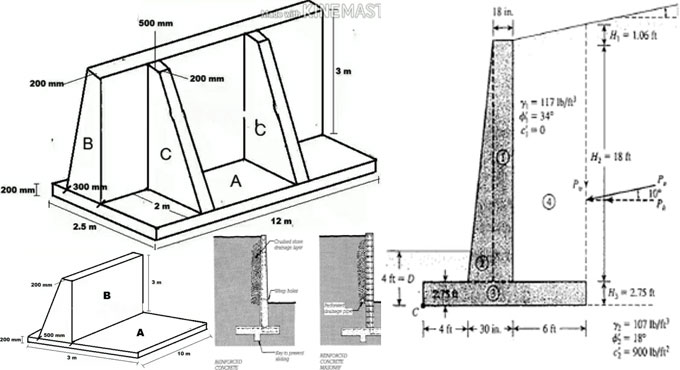How to work the volume of concrete in a retaining wallThis construction video will provide some useful tips on computing the volume of concrete for retaining wall.

Retaining wall is defined as a rigid wall that is designed and constructed to withstand lateral pressure of liquid, earth filling, sand or other coarse materials which it is holding back.

Suppose, a retaining wall is segregated into two sections, section A is taken as base slab and section B is taken as the stem of retaining wall.

Therefore, the volume of retaining wall is determined with the following formula :-

Volume of retaining wall = Volume of base slab + Volume of Stem

Volume of base slab = length x breadth x height

= 10 x 3 x 0.2 (after converting 200 mm to meter) = 6m3

As the stem is a trapezoid, the following formula is used to calculate it’s volume :-

Volume of stem = [{a+b)/2} x h] x l

After putting the values, we get :-

= [{(0.5 + 0.2)/2} x 3] x 10 = 21m3

Therefore, total volume of retaining wall = 6 + 21 = 27m3

Therefore, the volume of concrete for the retaining wall = 27m3

If the retaining wall is segregated into three parts like part A, part B and part C. Part A is taken as the base slab, part B is taken as the stem and part C is taken as the counterfort of the retaining wall.

Therefore, the volume of retaining wall = Volume of base slab + volume of stem + volume of counterfort

= Volume of A + Volume of B + Volume of C

To learn the remaining calculation process, go through the following video tutorial.

Video Source: Nice engineering